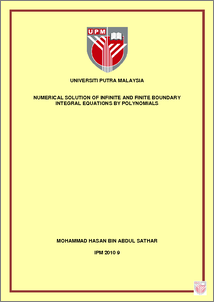Simple Search:

# Numerical Solution of Infinite and Finite Boundary Integral Equations by Polynomials

## Citation

Abdul Sathar, Mohammad Hasan (2010) Numerical Solution of Infinite and Finite Boundary Integral Equations by Polynomials. Masters thesis, Universiti Putra Malaysia.

## Abstract / Synopsis

The Fredholm Integral equation of the form g(s)=f(s) + ∫_a^b▒k(s,t)g(t)dt where k(s,t) is a regular kernel on D ={(s,t): a ~ s,t ~ b} and /(s) is a continuous function defined on [a,b] and the unknown function g(s) is to be determined, are encountered in many problems of ordinary differential equations and mathematical physics. Unfortunately in many cases the equation (I) cannot be solved in the closed form. Therefore, numerical method is applied to solve the integral equation (I). To approximate the integral equation (IE) (I), we usually choose a finite dimensional family of functions that is believed to contain a function g, (s) close to the true solutiong g(s). The desired approximate solution g,(s) is selected by forcing it to satisfy equation (I). There are various means in which g,(s) can be said to satisfy equation (I) approximately, and this leads to different type of methods. The most popular and powerful tools are Collocation and Galerkin methods. If the limit of integration in (I) is infinite and corresponding functions belong to certain class of functions then equation (I) is called infinite boundary integral equations (!BIEs). Many problems of electromagnetic, scattering problems and boundary integral equations lead to !BIEs of the second kind, g(s) =/(s) +4rk(s,t)g(t)dt, (2) g(s) =/(s) + 4 I k(s,t)g(t)dt. (3) In this thesis, we have developed Galerkin method with Laguerre polynomials for Eq. (2) on the interval [0,(0) and Galekin method with Hermite polynomials for Eq. (3) on the intetval (-00,00) to get the approximate solution. We have also solved equation (I) numerically using Collocation method based on Legendre polynomials on the interval [-1,1]. Existence of the solution for Eq. (2) and (3) and exactness of the approximate method for Eq. (I) are given. These developments gave good fit (even for small n) to the exact solution g(s) for finite and !BIEs (1)-(3). Maple software I I is used to obtain the approximate solution for Eq. (I )-(3) and the results show good convergence for finite and infinite interval for small n.

## Download FilePreview
PDF
IPM_2010_9_F.pdf

Download (392kB)

## Additional Metadata

Item Type: Thesis (Masters) Mathematical physics Infinite Finite element method IPM 2010 9 Institute for Mathematical Research Haridan Mohd Jais 09 May 2013 11:49 27 May 2013 16:02 http://psasir.upm.edu.my/id/eprint/19688 View Download Statistic

### Actions (login required)View Item